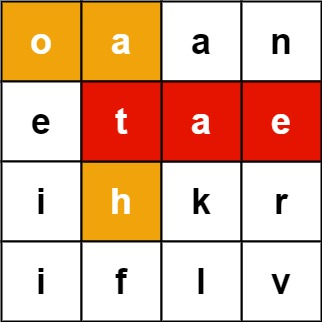# LeetCode-Word Search II Solution

#### 问题：

Given an `m x n` `board` of characters and a list of strings `words`, return all words on the board.

Each word must be constructed from letters of sequentially adjacent cells, where adjacent cells are horizontally or vertically neighboring. The same letter cell may not be used more than once in a word.

Example 1:Input:

``` board = [["o","a","a","n"],["e","t","a","e"],["i","h","k","r"],["i","f","l","v"]], words = ["oath","pea","eat","rain"]
```

Output:

``` ["eat","oath"]
```

Example 2:Input:

``` board = [["a","b"],["c","d"]], words = ["abcb"]
```

Output:

``` []
```

Constraints:

• `m == board.length`
• `n == board[i].length`
• `1 <= m, n <= 12`
• `board[i][j]` is a lowercase English letter.
• `1 <= words.length <= 3 * 10`4
• `1 <= words[i].length <= 10`
• `words[i]` consists of lowercase English letters.
• All the strings of `words` are unique.

#### 解答：

```class TrieNode():
def __init__(self):
self.children = collections.defaultdict(TrieNode)
self.isWord = False
class Trie():
def __init__(self):
self.root = TrieNode()

def insert(self, word):
node = self.root
for w in word:
node = node.children[w]
node.isWord = True

class Solution:
def findWords(self, board: List[List[str]], words: List[str]) -> List[str]:
res = []
trie = Trie()
node = trie.root
for w in words:
trie.insert(w)
for i in range(len(board)):
for j in range(len(board)):
self.dfs(board, node, i, j , "", res)
return res

def dfs(self, board, node, i, j, path, res):
if node.isWord:
res.append(path)
node.isWord = False

if i < 0 or i >= len(board) or j<0 or j>=len(board):
return

char = board[i][j]
node = node.children.get(char)
if not node:
return
board[i][j] = "#"
self.dfs(board, node, i+1, j, path+char, res)
self.dfs(board, node, i-1, j, path+char, res)
self.dfs(board, node, i, j-1, path+char, res)
self.dfs(board, node, i, j+1, path+char, res)
board[i][j] = char
```

#### 参考及引用

Python-dfs-solution-(directly-use-Trie-implemented).